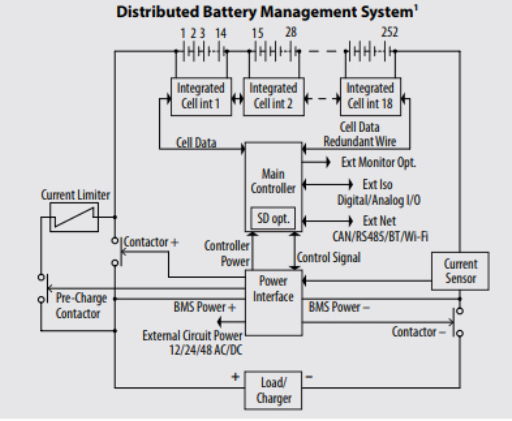Tel:400-803-9333
• Welcome to Microhm

#Position：Home » Applications

# Application of Resistors

Writer：万利隆电子 Page View：Date：2018-08-31
Resistors are passive electrical components helping control the flow of current in a circuit. A high resistance means less current is available for a given voltage. Inside a resistor, electrons collide with ions, slowing the flow of electricity and lowering the current while producing heat.

Timing and Frequency
A resistor connected to a capacitor provides a timing source in many circuit designs, electronic sirens light flashers and many other circuits depend on this feature. The capacitor,  holding electric charge like a cup holds water, takes a certain amount of time to fill with current, and the resistor determines how fast the capacitor is filled up. If you multiply the ohm value of a resistor by the farad value of a capacitor, you get a time value measured in seconds; as resistance increases, the circuit’s time period also increases.

Transistors and LEDs
Transistors and LEDs are sensitive to electrical current. A resistor of the correct value, placed in the circuit, allows transistors, LEDs and other semiconductor components to function in the current range that suits them best. As too much current will destroy them, but too little prevents them from working properly.Resistors for Heating
When converting electrical energy into heat, resistors make good heating elements for heaters, toasters, electric stoves and similar devices. Traditional light bulbs work because the very high temperature from their resistance turns a metal filament white-hot, producing light. A formula, P = I2 * R, where P is heating power in watts, I is current in amps, and R is resistance in ohms, determines the amount of heat given off by a resistor.

Voltage Divider
A voltage divider is a “daisy chain” of resistors connected together one after the other to form a series circuit. If the resistors are all with the same value, the voltage drop across each is equal. Otherwise it is a proportion determined by the resistance of each resistor and the total resistance of all the resistors in the divider. Voltage dividers are useful for components that need to operate at a lesser voltage than that supplied by the input.
User Control of Circuit Functions

Some resistors are variable by setting their resistance through sliding a slider or turning a knob. Varying resistance changes the amount of current flowing in a circuit. Variable resistor can be used to control the loudness of an amplifier, the speed of a motor, or the pitch of a musical tone.
Keywords：